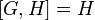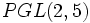# Difference between revisions of "A5 in S5"

This article is about a particular subgroup in a group, up to equivalence of subgroups (i.e., an isomorphism of groups that induces the corresponding isomorphism of subgroups). The subgroup is (up to isomorphism) alternating group:A5 and the group is (up to isomorphism) symmetric group:S5 (see subgroup structure of symmetric group:S5).
The subgroup is a normal subgroup and the quotient group is isomorphic to cyclic group:Z2.
VIEW: Group-subgroup pairs with the same subgroup part | Group-subgroup pairs with the same group part| Group-subgroup pairs with the same quotient part | All pages on particular subgroups in groups

## Definition

We define$G$ as symmetric group:S5 -- for concreteness, the symmetric group on the set$\{ 1,2,3,4,5 \}$.$H$ is alternating group:A5 -- the subgroup of$G$ comprising even permutations. A criterion for a permutation to be even, based on cycle decomposition, is that the number of cycles of even length should be even.

## Arithmetic functions

Function Value Explanation
order of group 120 Order is$5! = 5 \cdot 4 \cdot 3 \cdot 2 \cdot 1 = 120$
order of subgroup 60 Order is$5!/2 = 120/2 = 60$
index of subgroup 2 For degree$n \ge 2$, the alternating group of degree$n$ has index two in the symmetric group of degree$n$, since it has two cosets: itself (the even permutations) and the odd permutations.

## Subgroup-defining functions

Subgroup-defining function Meaning in general Why it takes this value GAP verification (set G := SymmetricGroup(5); H := AlternatingGroup(5);)
derived subgroup (also called commutator subgroup) subgroup generated by commutators Since the quotient is abelian, it contains the derived subgroup. Further,$H$ is simple non-abelian, so the derived subgroup cannot be smaller. H = DerivedSubgroup(G); using DerivedSubgroup
hypocenter stable member of lower central series (transfinite if necessary)$H$ is the derived subgroup, and$[G,H] = H$
perfect core stable member of derived series (transfinite if necessary); alternatively, join of all perfect subgroups$H$ is perfect, and the only bigger subgroup is$G$, which is not perfect because$[G,G] = H$
Jacobson radical intersection of all maximal normal subgroups$H$ is the unique maximal normal subgroup ($G$ is a one-headed group). This can also be seen from the fact that it is simple, so no other maximal normal subgroup can intersect it nontrivially, and it has no normal complement, so no maximal normal subgroup can intersect it nontrivially.
socle join of all minimal normal subgroups It is the unique minimal normal subgroup ($G$ is a monolithic group). H = Socle(G); using Socle
3-Sylow closure normal closure of any 3-Sylow subgroup Any 3-Sylow subgroup lies within$H$. Since$H$ is a minimal normal subgroup, it must be the normal closure of that subgroup.
5-Sylow closure normal closure of any 5-Sylow subgroup Any 5-Sylow subgroup lies within$H$. Since$H$ is a minimal normal subgroup, it must be the normal closure of that subgroup.

## Description in alternative interpretations of the whole group

Interpretation of$G$ Corresponding interpretation of$H$
Symmetric group of degree five. Alternating group of degree five.$PGL(2,5)$, the projective general linear group of degree two over field:F5$PSL(2,5)$, the projective special linear group of degree two over field:F5. In other words, the cosets mod the center of the linear transformations of determinant 1.$P\Gamma L(2,4)$, the projective semilinear group of degree two over field:F4.$PGL(2,4)$ (same as$PSL(2,4)$). In other words, the cosets mod scalars of the transformations that are actually linear, not merely semilinear.

## GAP implementation

### Finding this subgroup inside the group as a black box

Here, a group$G$ that we know to be isomorphic to the symmetric group of degree five is given, and we need to locate in that the alternating group of degree four. Different ways of constructing/locating this subgroup are given below.

Description Functions used
DerivedSubgroup(G) DerivedSubgroup
Socle(G) Socle

To assign$H$ to any of these, do H := followed by that. For instance:

H := DerivedSubgroup(G);

### Constructing the group and the subgroup

Because of GAP's native implementation of symmetric groups, this can be easily achieved using SymmetricGroup and AlternatingGroup:

gap> G := SymmetricGroup(5);;
gap> H := AlternatingGroup(5);;

Note that double semicolons have been used to suppress confirmatory output, but you may prefer to use single semicolons.